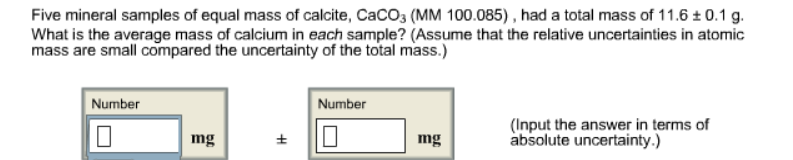# Problem: Five mineral samples of equal mass of calcite, CaCO3 (MM 100.085), had a total mass of 11.6 ± 0.1 g. What is the average mass of calcium in each sample? (Assume that the relative uncertainties in atomic mass are small compared the uncertainty of the total mass.)

###### FREE Expert Solution
89% (434 ratings)###### Problem Details

Five mineral samples of equal mass of calcite, CaCO3 (MM 100.085), had a total mass of 11.6 ± 0.1 g. What is the average mass of calcium in each sample? (Assume that the relative uncertainties in atomic mass are small compared the uncertainty of the total mass.)